Z-Tree
 What is Z-Tree

Z-Tree is a new data structure for sorting, key-value mapping and multiple other purposes.
Sorting with Z-Tree is not based on comparison and the time complexity of sorting with Z-Tree is O(n). Z-Tree is capable of sorting files of several GB in memory.
For key-value mapping, the time complexity of adding a key/value or finding a value by key is O(1).

Bit Values
In Z-Tree, all keys will be distinguished by bit values.
The following table shows some example keys and their bit values. In the following descriptions, the ASCII values instead of the UNICODE values will be used to make the demonstration simple.
 Keys Bit Values 1 00110001 a 01100001 2 00110010 ab 01100001 01100010 long key 01101100 01101111 01101110 01100111 00100000 01101011 01100101 01111001

Z-Tree Components
Z-Tree includes three kinds of nodes, Key Node, Branch Node and Value Node.
Both Key Node and Branch Node include a pointer pointing to the Value Nodes associated with the key. The Value Nodes are optional and may vary in different applications. The Value Node will not be discussed in detail here.

Key Node
Key Node represents multiple continuous bits. Key Node includes a bit buffer together with the start bit index and the end bit index to represent multiple continuous bits.
Key Node also includes a pointer pointing to the child Key Node or Branch Node and another pointer pointing to the Value Nodes. Figure 1 shows a Key Node with 6 bits "111011" (the pointer to the Value Nodes is not shown). Figure 2 shows the Key Node definition in C/C++.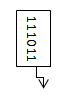Figure 1: Z-Tree Key Node with 6 bits "111011"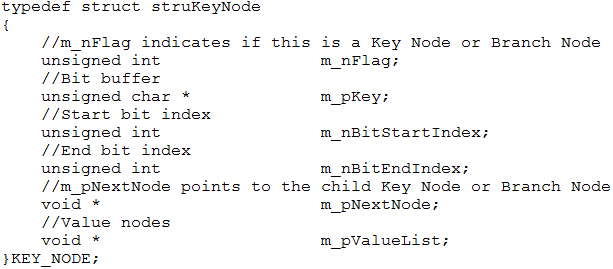Figure 2: Z-Tree Key Node definition in C/C++

Branch Node
Branch Node includes two pointers representing bit 0 and bit 1. The first pointer (for bit 0) points to the left child Key Node or Branch Node and the second pointer (for bit 1) points to the right child Key Node or Branch Node. Branch Node also includes a pointer pointing to the Value Nodes. Figure 3 shows a Branch Node (the pointer to the Value Nodes is not shown). Figure 4 shows the Branch Node definition in C/C++.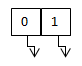Figure 3: Z-Tree Branch NodeFigure 4: Z-Tree Branch Node definition in C/C++

Z-Tree Operations
Z-Tree includes three kinds of operations, adding a key (and associated value) into Z-Tree, finding a key (and associated value) from Z-Tree and traversing Z-Tree to sort over the keys.
Removing a key (and associated value) will not be discussed here.

Add a Key (and Associated Value) into Z-Tree
When a new key (and associated value) is added into Z-Tree, Z-Tree will perform a loop to compare the bit values of the incoming key and the bit values of the Z-Tree nodes until reaches the end of Z-Tree or the incoming key.
If the current Z-Tree node is a Branch Node and the current bit value of the incoming key is 0, Z-tree will go to the left child node.
If the current Z-Tree node is a Branch Node and the current bit value of the incoming key is 1, Z-tree will go to the right child node.
If the current Z-Tree node is a Key Node and the bit values of the Key Node in Z-Tree match the current bit values of the incoming key, Z-tree will go to the child node.
If the current Z-Tree node is a Key Node and the bit values of the Key Node don't match the current bit values of the incoming key, the Key Node in Z-Tree will be split at the first different bit. If the first bit is different, the Key Node in Z-Tree will be split into one Branch Node and one Key Node. If the first different bit is the last bit, the Key Node in Z-Tree will be split into one Key Node and one Branch Node. If the first different bit is in the middle of the Z-Tree Key Node, the Key Node will be split into one Key Node, one Branch Node and another Key Node. After that, Z-Tree will continue with the loop.
If after reaching the end of the Z-Tree, there is still extra bits in the incoming key, Z-Tree will create a new Key Node and append it to the end of Z-Tree.
The value, if there is, will be added to the value list of the last Key Node or Branch Node.
The time complexity of adding a key (and associated value) is always O (1) because the time complexity depends on the length of bits and is irrelevant to the key number (n).

Examples of Adding Keys (and Associated Values) to Z-Tree
Here are some examples about adding keys (and associated values) to Z-Tree.
Figure 5 shows Z-Tree after adding a key "1" (00110001).
Figure 6 show Z-Tree after adding another key "a" (01100001). Since the second bit is different, the Key Node will be split and a Branch Node will be inserted at the second bit. Key "1" (00110001) will go to the left child tree (bit 0) and key "a" (01100001) will go to the right child tree (bit 1).
Figure 7 shows Z-Tree after adding another key "2" (00110010). This time the seventh bit is different and will be split.
Figure 8 shows Z-Tree after adding another key "ab" (0110000101100010). A new Key Node will be created at the end of Z-Tree.Figure 5: Z-Tree after adding keys "1" (00110001)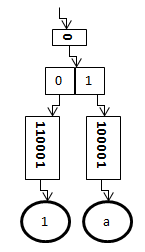Figure 6: Z-Tree after adding keys "1" (00110001) and "a" (01100001)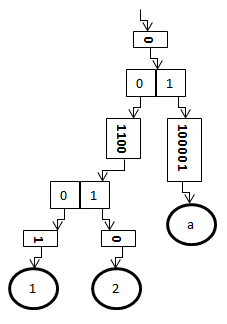Figure 7: Z-Tree after adding keys "1" (00110001), "a" (01100001) and "2" (00110010)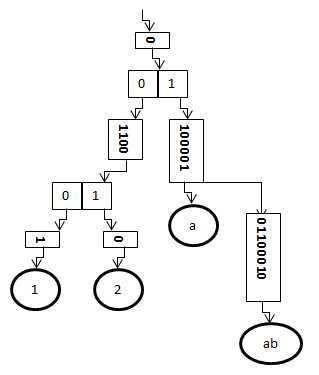Figure 8: Z-Tree after adding keys "1" (00110001), "a" (01100001), "2" (00110010) and "ab" (0110000101100010)

Find a Key (and associated value) from Z-Tree
When trying to find a key (and associated value) in Z-Tree. Z-Tree will perform a loop to compare the bit values of the incoming key and the bit values of the Z-Tree nodes until reaches the end of Z-Tree or the incoming key.
If the current Z-Tree node is a Branch Node and the current bit value of the incoming key is 0, Z-tree will go to the left child node.
If the current Z-Tree node is a Branch Node and the current bit value of the incoming key is 1, Z-tree will go to the right child node.
If the current Z-Tree node is a Key Node and the bit values of the Key Node match the current bit values of the incoming key, Z-tree will go to the child node.
If the current Z-Tree node is a Key Node and the bit values of the Key Node don't match the current bit values of the incoming key, Z-tree will return null.
When Z-Tree reaches the end of the incoming key, the value list of the last matching Z-Tree Key Node or Branch Node will be returned.
The time complexity of finding a key (and associated value) in Z-Tree is always O (1) since there is no collision between the bit values of different keys.
Figure 9 shows how to find a key "1" (and the associated value) in Z-Tree following the bit values of the key "1" (00110001).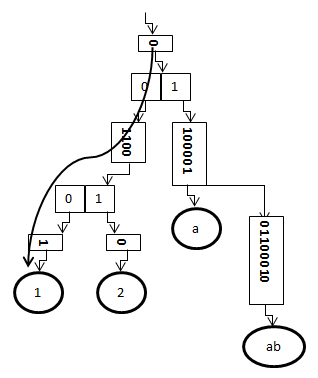Figure 9: Find key "1" (00110001) and the associated values in Z-Tree

Traverse Z-Tree to Sort Over the Keys
Figure 10 shows that the keys in Z-Tree are already sorted automatically. One can sort over the keys by traversing the Key Node and Branch Node recursively. The following steps show how to sort over the keys in ascending order.
If the current node has Value Nodes, output the values.
If the current node is a Branch Node, traverse the left child tree and then traverse the right child tree.
If the current node is a Key Node and has a child node, go on to traverse the child tree.
The time complexity of sorting with Z-Tree is O (n) which is the fastest among all sorting algorithms.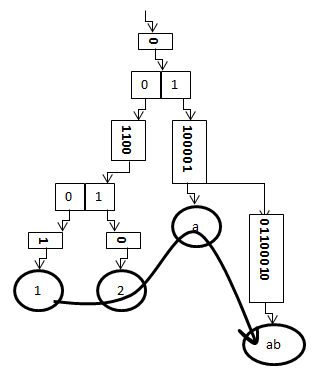Figure 10: The keys in Z-Tree are sorted automatically

Z-Tree has many advantages when compared with hash table and other data structures.
1. Z-Tree will distinguish keys by bit values instead of hash code. Since the different keys must have different bit values, there is no collision between different keys. The time complexity of adding/finding a key in Z-Tree is always O (1).
2. Z-Tree can grow automatically. There is no need to worry about the bucket size. By comparison, hash table needs to copy keys/values from one bucket to another bucket when it is growing.
3. Since the keys in Z-Tree are sorted automatically, one can use Z-Tree to sort millions of keys. The time complexity of sorting with Z-Tree is O (n) which is the fastest among all sorting algorithms.
4. When two keys have the same prefix, they can share the same Key Nodes and Branch Nodes for the prefix. For example, for the two keys, "Hello Tom" and "Hello Jack", the prefix "Hello " will be saved in the same Key Nodes or Branch Nodes. This feature can help to reduce the memory usage. While loading a file into Z-Tree, the memory size allocated for Z-Tree may be even less than the file size. That is why one can use Z-Tree to sort files of several GB.
5. Z-Tree can be used to find all keys started with a prefix conveniently. For example, one can find all keys started with "Hello" in Z-Tree.
6. Z-Tree can also be used to save binary keys and values.